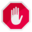## Name

ST_Length — Returns the 2D length of a linear geometry.

## Synopsis

`float ST_Length(`geometry a_2dlinestring`)`;

`float ST_Length(`geography geog, boolean use_spheroid=true`)`;

## Description

For geometry types: returns the 2D Cartesian length of the geometry if it is a LineString, MultiLineString, ST_Curve, ST_MultiCurve. For areal geometries 0 is returned; use ST_Perimeter instead. The units of length is determined by the spatial reference system of the geometry.

For geography types: computation is performed using the inverse geodesic calculation. Units of length are in meters. If PostGIS is compiled with PROJ version 4.8.0 or later, the spheroid is specified by the SRID, otherwise it is exclusive to WGS84. If `use_spheroid=false`, then the calculation is based on a sphere instead of a spheroid.

Currently for geometry this is an alias for ST_Length2D, but this may change to support higher dimensions.Changed: 2.0.0 Breaking change -- in prior versions applying this to a MULTI/POLYGON of type geography would give you the perimeter of the POLYGON/MULTIPOLYGON. In 2.0.0 this was changed to return 0 to be in line with geometry behavior. Please use ST_Perimeter if you want the perimeter of a polygonFor geography the calculation defaults to using a spheroidal model. To use the faster but less accurate spherical calculation use ST_Length(gg,false);This method implements the OGC Simple Features Implementation Specification for SQL 1.1. s2.1.5.1This method implements the SQL/MM specification. SQL-MM 3: 7.1.2, 9.3.4

Availability: 1.5.0 geography support was introduced in 1.5.This method is also provided by SFCGAL backend.

## Geometry Examples

Return length in feet for line string. Note this is in feet because EPSG:2249 is Massachusetts State Plane Feet

```SELECT ST_Length(ST_GeomFromText('LINESTRING(743238 2967416,743238 2967450,743265 2967450,
743265.625 2967416,743238 2967416)',2249));

st_length
---------
122.630744000095

--Transforming WGS 84 LineString to Massachusetts state plane meters
SELECT ST_Length(
ST_Transform(
ST_GeomFromEWKT('SRID=4326;LINESTRING(-72.1260 42.45, -72.1240 42.45666, -72.123 42.1546)'),
26986
)
);

st_length
---------
34309.4563576191
```

## Geography Examples

Return length of WGS 84 geography line

```-- the default calculation uses a spheroid
SELECT ST_Length(the_geog) As length_spheroid,  ST_Length(the_geog,false) As length_sphere
FROM (SELECT ST_GeographyFromText(
'SRID=4326;LINESTRING(-72.1260 42.45, -72.1240 42.45666, -72.123 42.1546)') As the_geog)
As foo;

length_spheroid  |  length_sphere
------------------+------------------
34310.5703627288 | 34346.2060960742
```